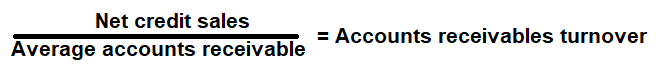# Accounts Receivable Turnover Calculator

Key in the Nets Sales and Accounts Receivable Values to the respective fields given below and then click Calculate to get the desired result.

# Accounts Receivable Turnover Ratio Calculator - Glossary:

Accounts Receivable Turnover Ratio: Shows the proportion of Net Sales to Average Account Receivable. In simple words, it helps a company to determine the average time it took to collect its money.

### Formula:### How to use this equation?

The values are commonly stated against accounts receivable under current assets in the balance sheet and net sales in the income statement. To use this ratio, divide net sales by its average accounts receivable.
Note:
Average Accounts Receivable (AR): Add accounts receivable of the current(ending) year and the accounts receivable of the previous (beginning) year, and then divide that value by 2.

Net Sales:
Total sales less returns and bad debts.

Account Receivable:
Account receivable is the net value of credit sales to be collected in future from its customers (Debtors).

Example:
Account receivable turnover ratio for a company with a net sales income of \$360,000 and average accounts receivable of \$30,000 is 12 times. It means that an average customer took 30 days to pay the company's credit sales. This metric helps a company to determine the average time it took to collect its money (AR) by dividing the Accounts Receivable Turnover Ratio/365days.